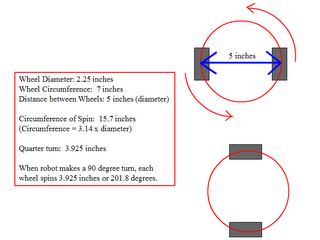We received this question: "If I want my tribot to turn 90 degrees, how come I have to code the motors to spin in opposite directions each for 180 (or a bit more) degrees? I mean, why 180 degrees if I am turning the robot 90 degrees?"

Click on the image at left. If you take the NXT stock wheels (2.25 inches in diameter) and calculate their circumference, you get approx. 7 inches. Now, assume those wheels are mounted on a bot as shown, with a distance of 5 inches between them and you want the bot to spin a quarter-turn in place (left wheel spins one direction, right wheel spins the opposite direction).

When the Bot makes a quarter turn, each wheel has traveled 3.925 inches (along the path indicated by the red circle). If 7 inches = 360 degrees, then a simple calculation shows that 3.925 inches is approx 201 degrees - not 90.

The bot as a whole has made a 90 degree turn, but the wheels have turned substantially more.

Did this help? Can someone verify my math and that I've done this correctly?

JimAnonymous said…
The fact that you have to turn the motors 180 degrees to turn your TriBot 90 degrees actually is a coincidence.

The amount of degrees you actually have to turn depends on the distance the wheels have to go to traverse a 90 degree circle.

This is:
Pi/2 * r

This may look a bit cryptic so I'll explain in detail. The first part is the 90 degrees angle in radians. To convert an angle from degrees to radians, just divide by 180 and multiply by Pi.

The second part is the radius, which is the distance between the two wheels divided by 2.

Let's take the TriBot example. the centers of its wheels are spaced 13 studs apart. A stud is 7.9mm wide, so the distance between the wheels is 10.3mm.

Now we need to know how many rotations the wheel should make to traverse 10.3mm. The circumference of the weels is 18.4mm, so traversing 10.3mm takes 56% of a full revolution, which is pretty close to 180 degrees (assuming there is no gear reduction between the motor and the wheel).Also, as the distance between the two wheels increases, you'll see a substantial jump in the number of degrees each individual motor must spin a wheel... try it out by building a bot with wheels about 10 inches apart...

JimAnonymous said…
yay... i was calculating tha same thing... TODAY! not joking :OAnonymous said…
Yes, Off coarse, you are talking about a 90 degree rotation of the whole NXT in a plane perpendicular to that of the rotation of the wheels. The NXT software only looks at rotation of the wheel shaft and not of any other rotation which is always a translation.

Regards, FranklinAnonymous said…
I was the one who submitted the question, so thanks for the answers. Yeah, it's been awhile since I've taken geometry. :)

Without using formulas we found a value of 192 degrees (for the motors) turns the tribot pretty close to 90 degrees.

Now, does friction or momentum also enter into the question? I mean, say the bot is travelling forward at some at 30 speed, then you tell it to turn 45 degrees right. What if it were travelling forward at 75 or 90 speed...?

I guess I can always experiment, but having the reason behind something does help--just as having the answer to my original question.

Thanks!Brian Davis said…
First suggestion: you're right, try it. Actual results are always preferable to estimations and theory.

Second, you made the observation that a wheel odometry count of 192° corresponds to a heading change of 90° (in, I assume, a spin turn, with both wheels going in opposite directions - it will be different for, say, a pivot turn with one wheel fixed!). That *should* be due to just the geometry of the platform (the wheel radius, any gearing, and the wheelbase (distance between the wheels), so the speed with which you go into the turn shouldn't make a difference, *IF* there is no wheel slip. But in practice... try it and see.

--
Brian DavisAnonymous said…
Some Math,

1) Distance between the Wheels is S
2) Diameter of the wheels is d

3) Circumference of Base Circle is pi * S

4) Circumference of Wheel is pi * d

When we devide 3) by 4) we get: N

N is de number of Wheel Turns required for 1 complete 360 degrees Base Turn.

pi * S S
N = ------ = ---
pi * d d

note: pi = 3.1415 falls out of the equation.

The above is only true when both wheels turn at the same time!!!

When only 1 wheel turns and the other stays fixed the Circumference of the Base Circle then becomes 2 * pi * S

(S becomes the radiant of the circle and not the diameter!)

2 * S
then N = -----
d

So , now one can easily calculate the number of wheel turns in any situation.

Regards from a Dutch Lego Fan,

FranklinAnonymous said…
Franklin again,

the fomula did not get across well,

here is another attempt:

N = S / d (when both wheels turn!)

N = (2 * S) / d (when only 1 wheel turns)

FranklinAnonymous said…
Please any body could share the source code to rotate the wheels in such a way.
Thanks.